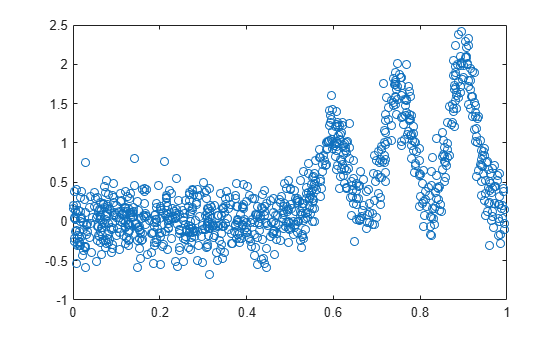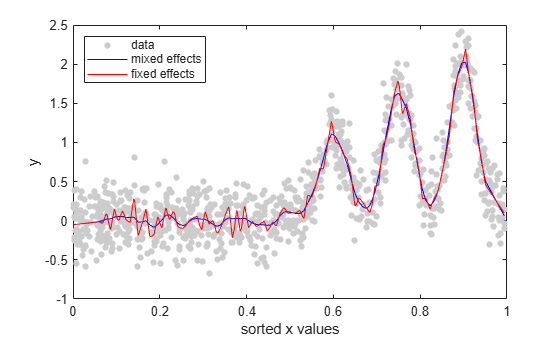# Fit Mixed-Effects Spline Regression

This example shows how to fit a mixed-effects linear spline model.

`load('mespline.mat');`

This is simulated data.

Plot $y$ versus sorted $x$.

```[x_sorted,I] = sort(x,'ascend'); plot(x_sorted,y(I),'o')```Fit the following mixed-effects linear spline regression model

`${y}_{i}={\beta }_{1}+{\beta }_{2}{x}_{i}+\sum _{j=1}^{K}{b}_{j}{\left({x}_{i}-{k}_{j}\right)}_{+}+{ϵ}_{i}$`

where ${k}_{j}$ is the $j$ th knot, and $K$ is the total number of knots. Assume that ${b}_{j}\sim N\left(0,{\sigma }_{b}^{2}\right)$ and $ϵ\sim N\left(0,{\sigma }^{2}\right)$.

Define the knots.

`k = linspace(0.05,0.95,100);`

Define the design matrices.

```X = [ones(1000,1),x]; Z = zeros(length(x),length(k)); for j = 1:length(k) Z(:,j) = max(X(:,2) - k(j),0); end```

Fit the model with an isotropic covariance structure for the random effects.

`lme = fitlmematrix(X,y,Z,[],'CovariancePattern','Isotropic');`

Fit a fixed-effects only model.

```X = [X Z]; lme_fixed = fitlmematrix(X,y,[],[]);```

Compare `lme_fixed` and `lme` via a simulated likelihood ratio test.

`compare(lme,lme_fixed,'NSim',500,'CheckNesting',true)`
```ans = Simulated Likelihood Ratio Test: Nsim = 500, Alpha = 0.05 Model DF AIC BIC LogLik LRStat pValue Lower Upper lme 4 170.62 190.25 -81.309 lme_fixed 103 113.38 618.88 46.309 255.24 0.68064 0.63784 0.72129 ```

The $p$-value indicates that the fixed-effects only model is not a better fit than the mixed-effects spline regression model.

Plot the fitted values from both models on top of the original response data.

```R = response(lme); figure(); plot(x_sorted,R(I),'o', 'MarkerFaceColor',[0.8,0.8,0.8],... 'MarkerEdgeColor',[0.8,0.8,0.8],'MarkerSize',4); hold on F = fitted(lme); F_fixed = fitted(lme_fixed); plot(x_sorted,F(I),'b'); plot(x_sorted,F_fixed(I),'r'); legend('data','mixed effects','fixed effects','Location','NorthWest') xlabel('sorted x values'); ylabel('y'); hold off```You can also see from the figure that the mixed-effects model provides a better fit to data than the fixed-effects only model.